# EECS 274 Computer Vision Model Fitting Fitting Choose

• Slides: 42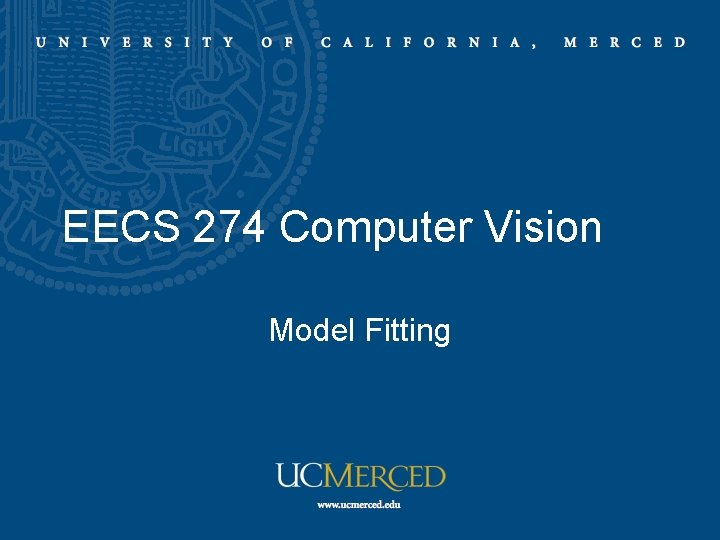EECS 274 Computer Vision Model Fitting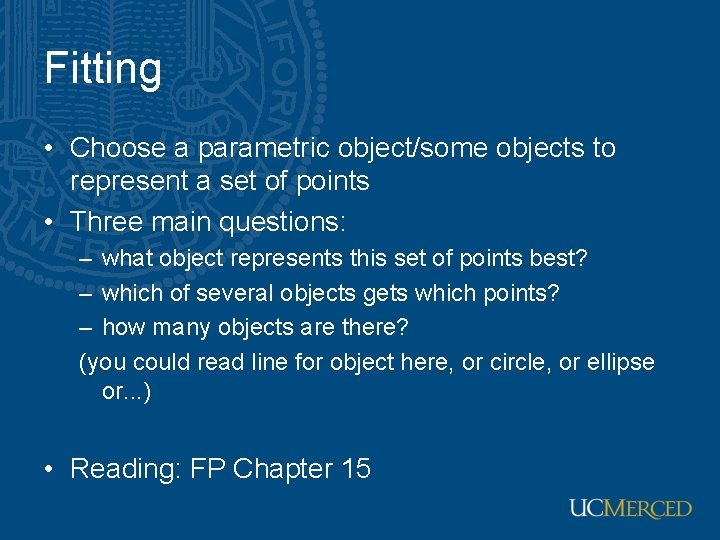Fitting • Choose a parametric object/some objects to represent a set of points • Three main questions: – what object represents this set of points best? – which of several objects gets which points? – how many objects are there? (you could read line for object here, or circle, or ellipse or. . . ) • Reading: FP Chapter 15Fitting and the Hough transform • Purports to answer all three questions – in practice, answer isn’t usually all that much help • We do for lines only • A line is the set of points (x, y) such that • Different choices of , d>0 give different lines • For any (x, y) there is a one parameter family of lines through this point, given by • Each point gets to vote for each line in the family; if there is a line that has lots of votes, that should be the line passing through the pointstokens votes • 20 points • 200 bins in each direction • # of votes is indicated by the pixel brightness • Maximum votes is 20 • Note that most points in the vote array are very dark, because they get only one vote.Hough transform • Construct an array representing , r • For each point, render the curve ( , r) into this array, adding one at each cell • Difficulties – Quantization error: how big should the cells be? (too big, and we cannot distinguish between quite different lines; too small, and noise causes lines to be missed) – Difficulty with noise • How many lines? – count the peaks in the Hough array • Who belongs to which line? – tag the votes • Hardly ever satisfactory in practice, because problems with noise and cell size defeat it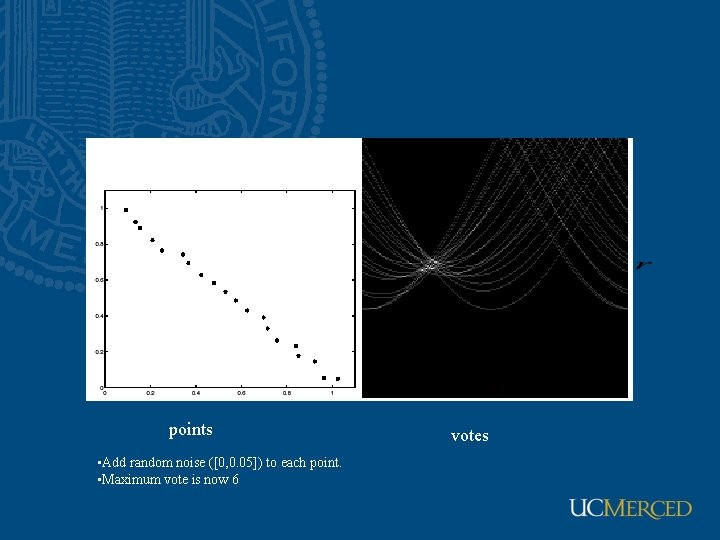points • Add random noise ([0, 0. 05]) to each point. • Maximum vote is now 6 votes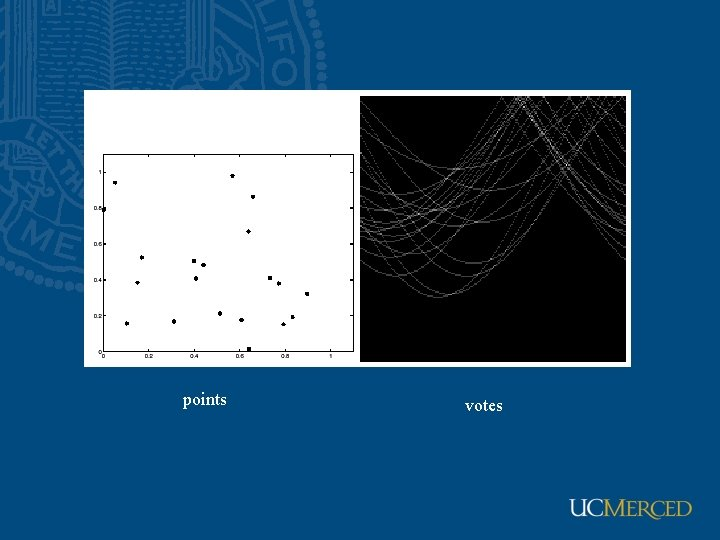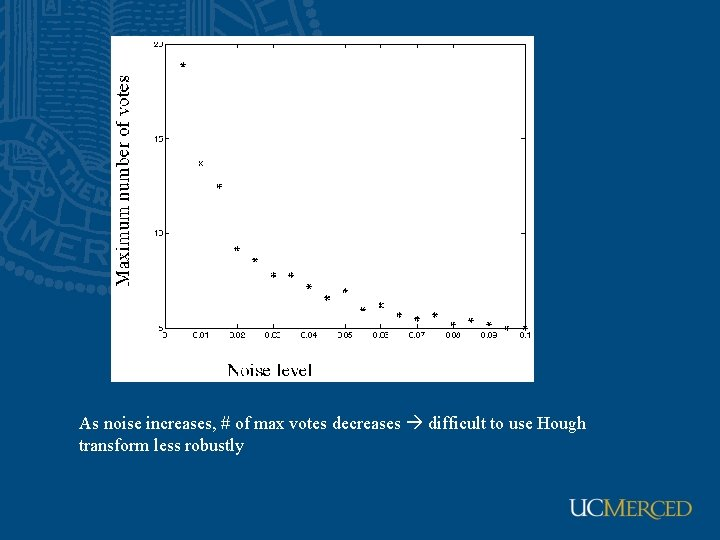As noise increases, # of max votes decreases difficult to use Hough transform less robustly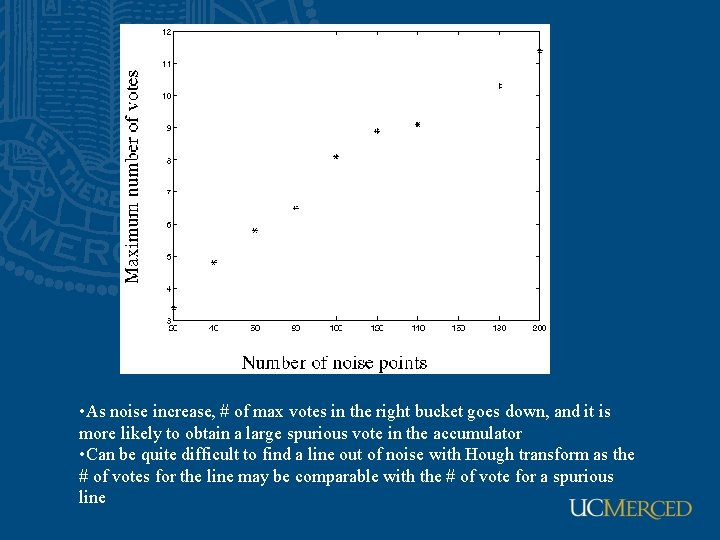• As noise increase, # of max votes in the right bucket goes down, and it is more likely to obtain a large spurious vote in the accumulator • Can be quite difficult to find a line out of noise with Hough transform as the # of votes for the line may be comparable with the # of vote for a spurious lineChoice of model Least squares but assumes error appears only in y Total least squaresWho came from which line? • Assume we know how many lines there are - but which lines are they? – easy, if we know who came from which line • Three strategies – Incremental line fitting – K-means – Probabilistic (later!)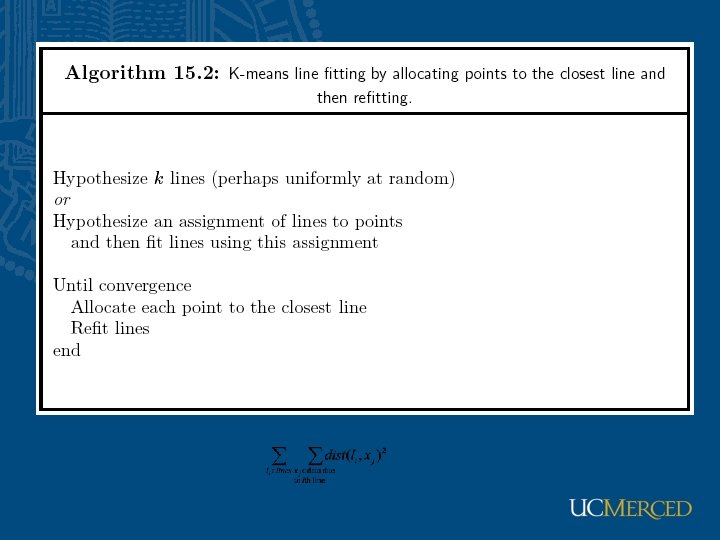Fitting curves other than lines • In principle, an easy generalisation – The probability of obtaining a point, given a curve, is given by a negative exponential of distance squared • In practice, rather hard – It is generally difficult to compute the distance between a point and a curveImplicit curves • (u, v) on curve, i. e. , ϕ(u, v)=0 • s=(dx, dy)-(u, v) is normal to the curveRobustness • As we have seen, squared error can be a source of bias in the presence of noise points – One fix is EM - we’ll do this shortly – Another is an M-estimator • Square nearby, threshold far away – A third is RANSAC • Search for good points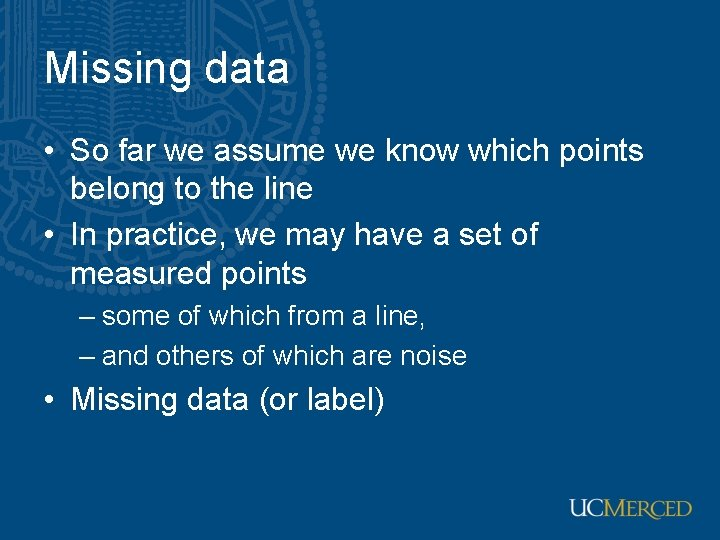Missing data • So far we assume we know which points belong to the line • In practice, we may have a set of measured points – some of which from a line, – and others of which are noise • Missing data (or label)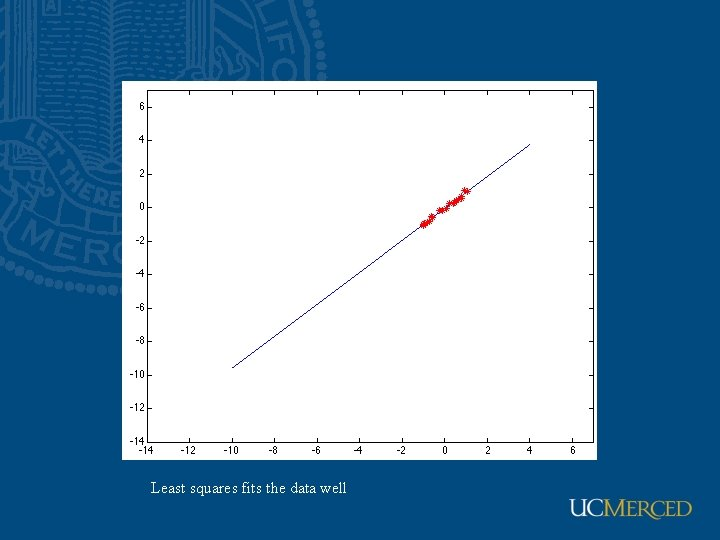Least squares fits the data wellSingle outlier (x-coordinate is corrupted) affects the least-squares resultSingle outlier (y-coordinate is corrupted) affects the least-squares result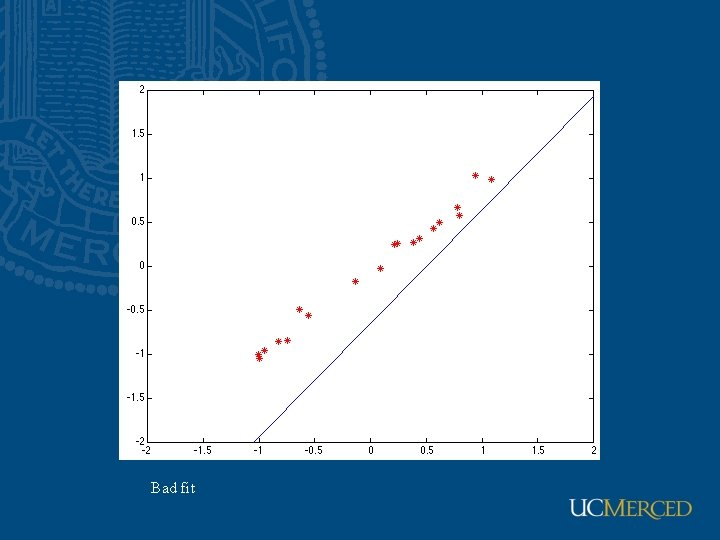Heavy tail, light tail • • • The red line represents a frequency curve of a long tailed distribution. The blue line represents a frequency curve of a short tailed distribution. The black line is the standard bell curve. .M-estimators • Often used in robust statistics A point that is several away from the fitted curve will have no effect on the coefficientsOther M-estimators • Defined by influence function • Nonlinear function, solved iteratively • Iterative strategy – Draw a subset of samples randomly – Fit the subset using least squares – Use the remaining points to see fitness • Need to pick a sensible σ, which is referred as scale • Estimate scale at each iteration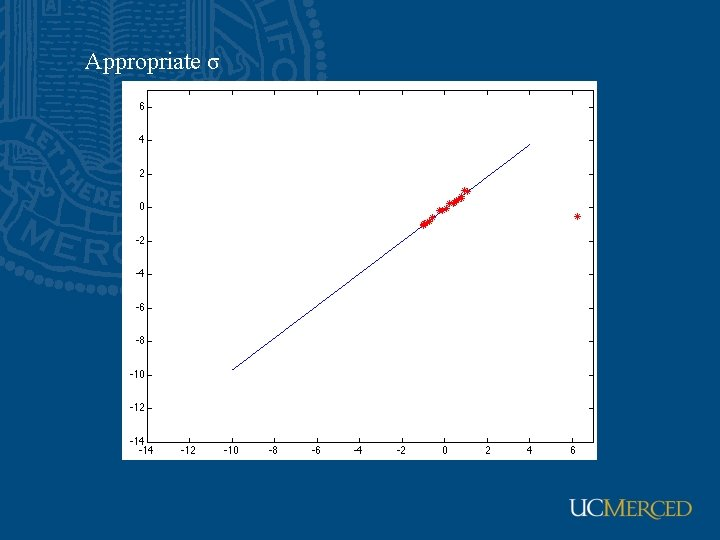Appropriate σsmall σ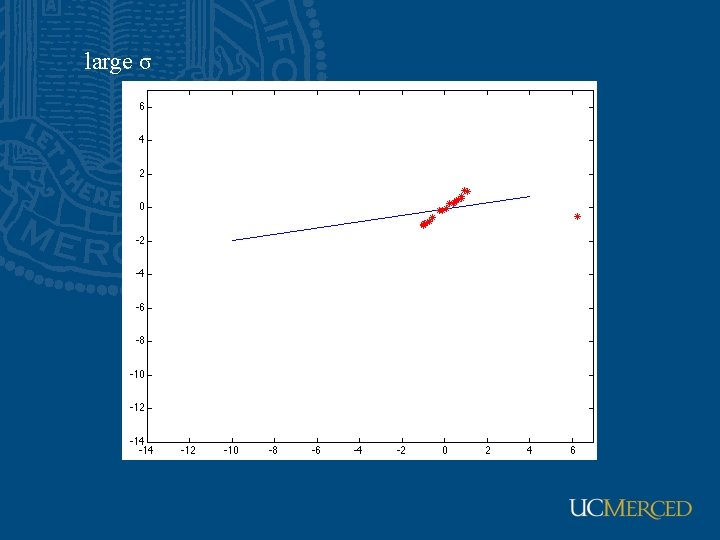large σ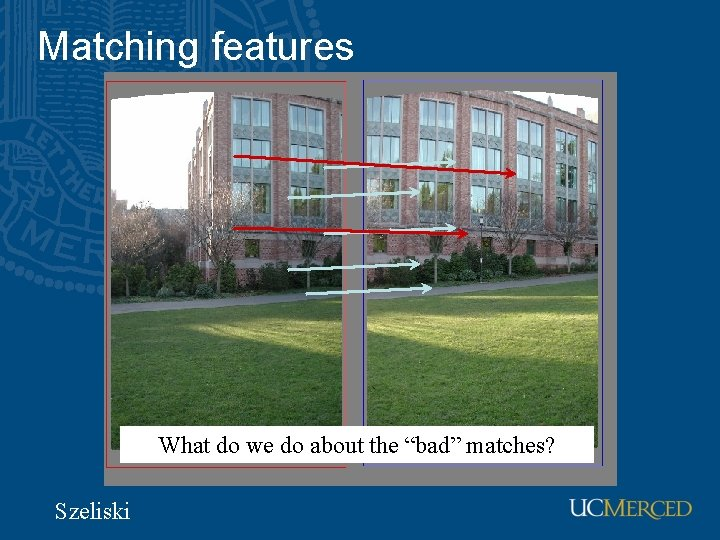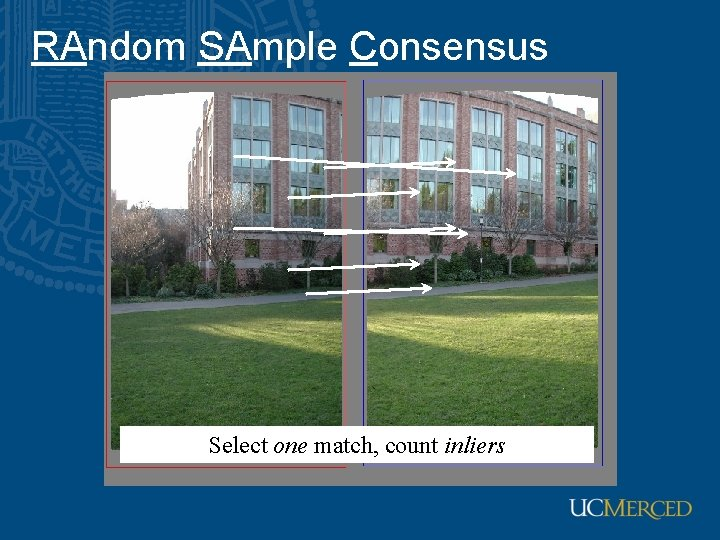RAndom SAmple Consensus Select one match, count inliersRAndom SAmple Consensus Select one match, count inliers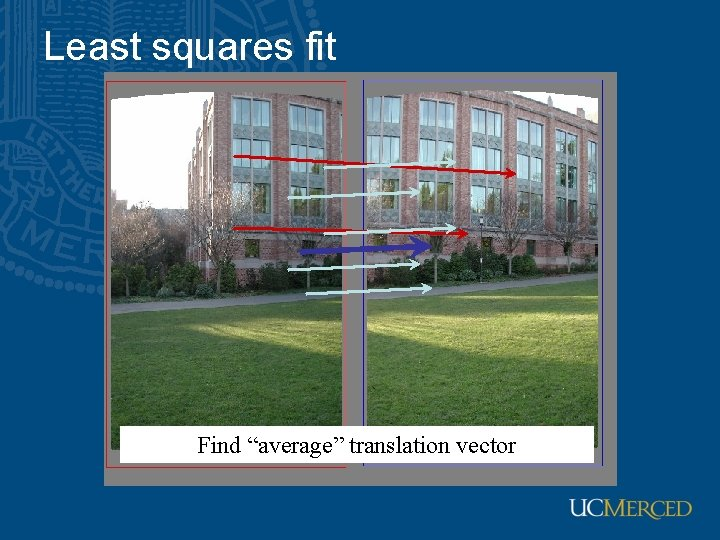Least squares fit Find “average” translation vectorRANSAC • Random Sample Consensus • Choose a small subset uniformly at random • Fit to that • Anything that is close to result is signal; all others are noise • Refit • Do this many times and choose the best • Issues – How many times? • Often enough that we are likely to have a good line – How big a subset? • Smallest possible – What does close mean? • Depends on the problem – What is a good line? • One where the number of nearby points is so big it is unlikely to be all outliers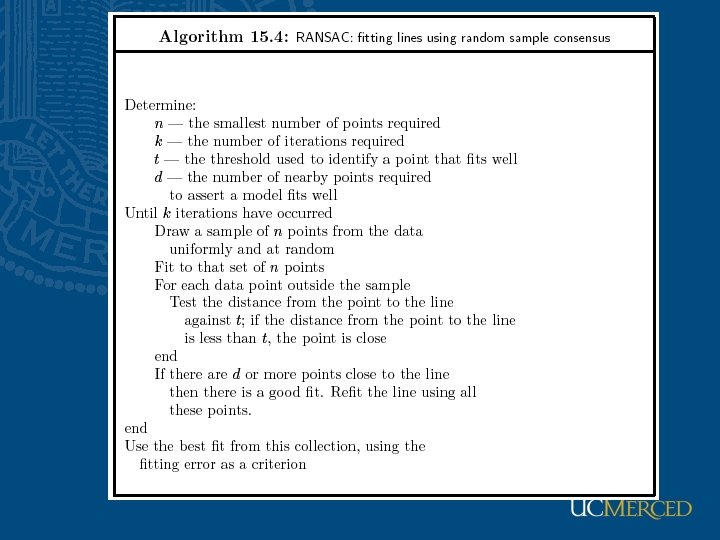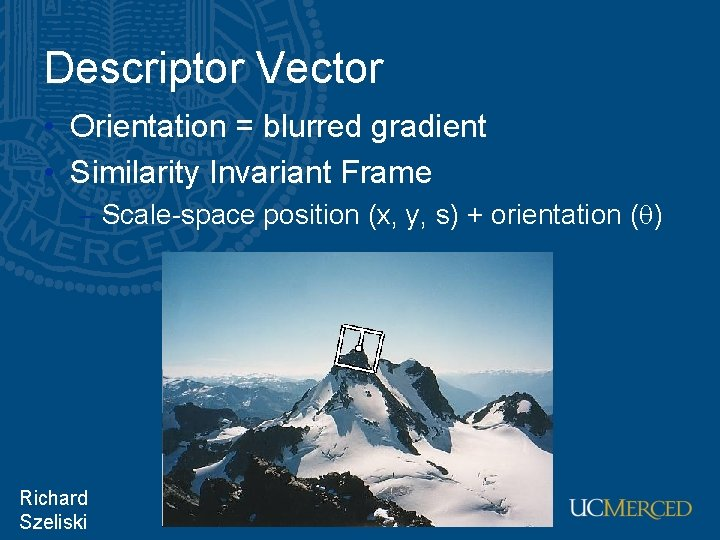Descriptor Vector • Orientation = blurred gradient • Similarity Invariant Frame – Scale-space position (x, y, s) + orientation ( ) Richard Szeliski Image Stitching 34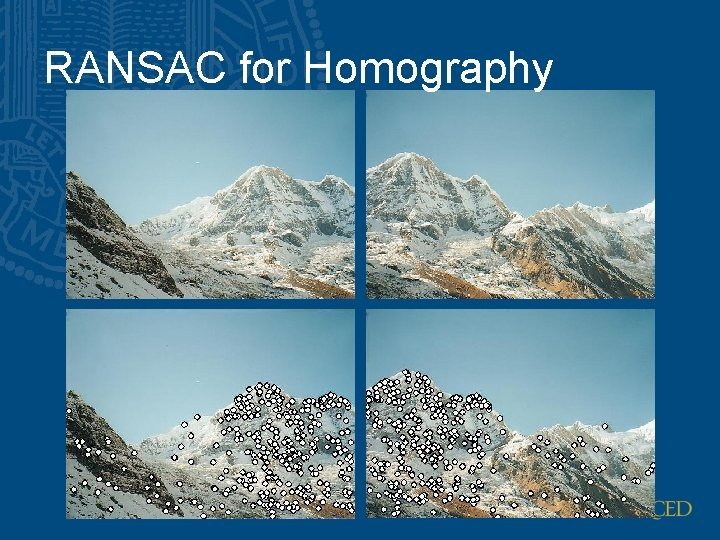RANSAC for HomographyRANSAC for HomographyRANSAC for HomographyProbabilistic model for verification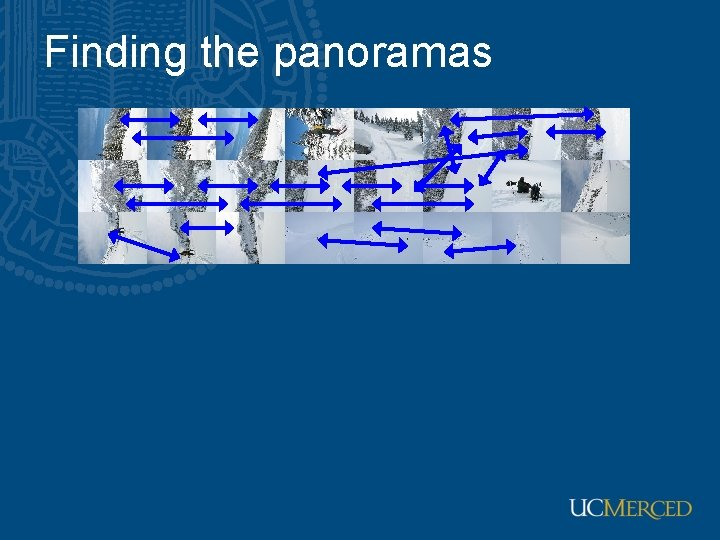Finding the panoramas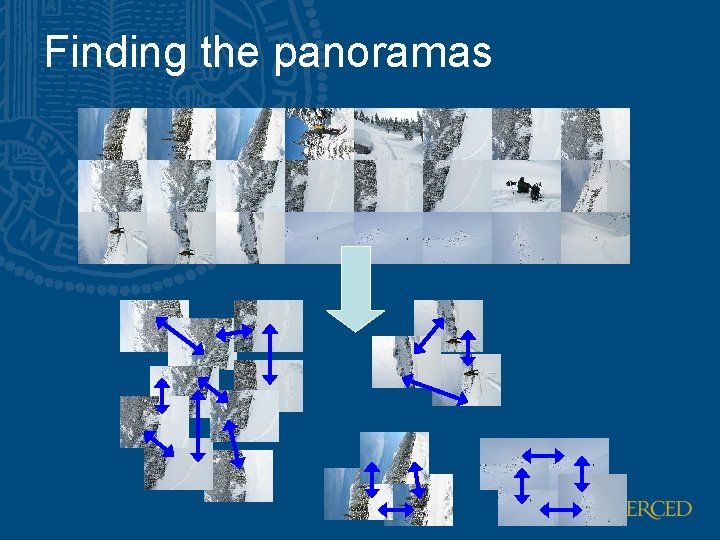Finding the panoramasFinding the panoramas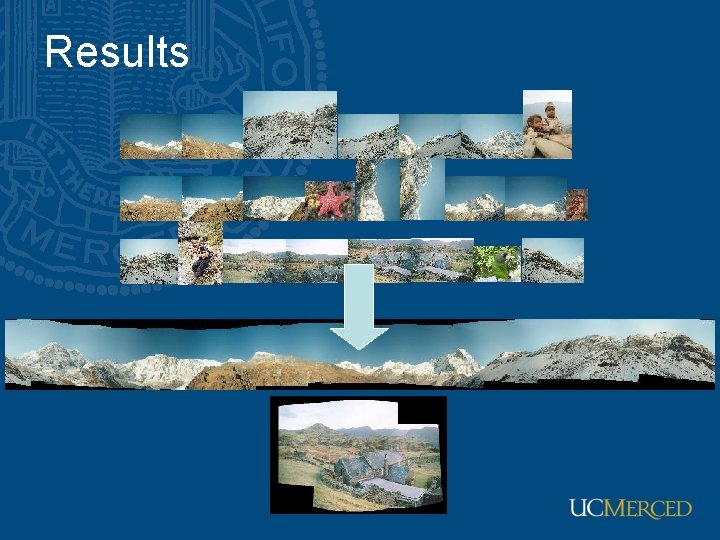Results